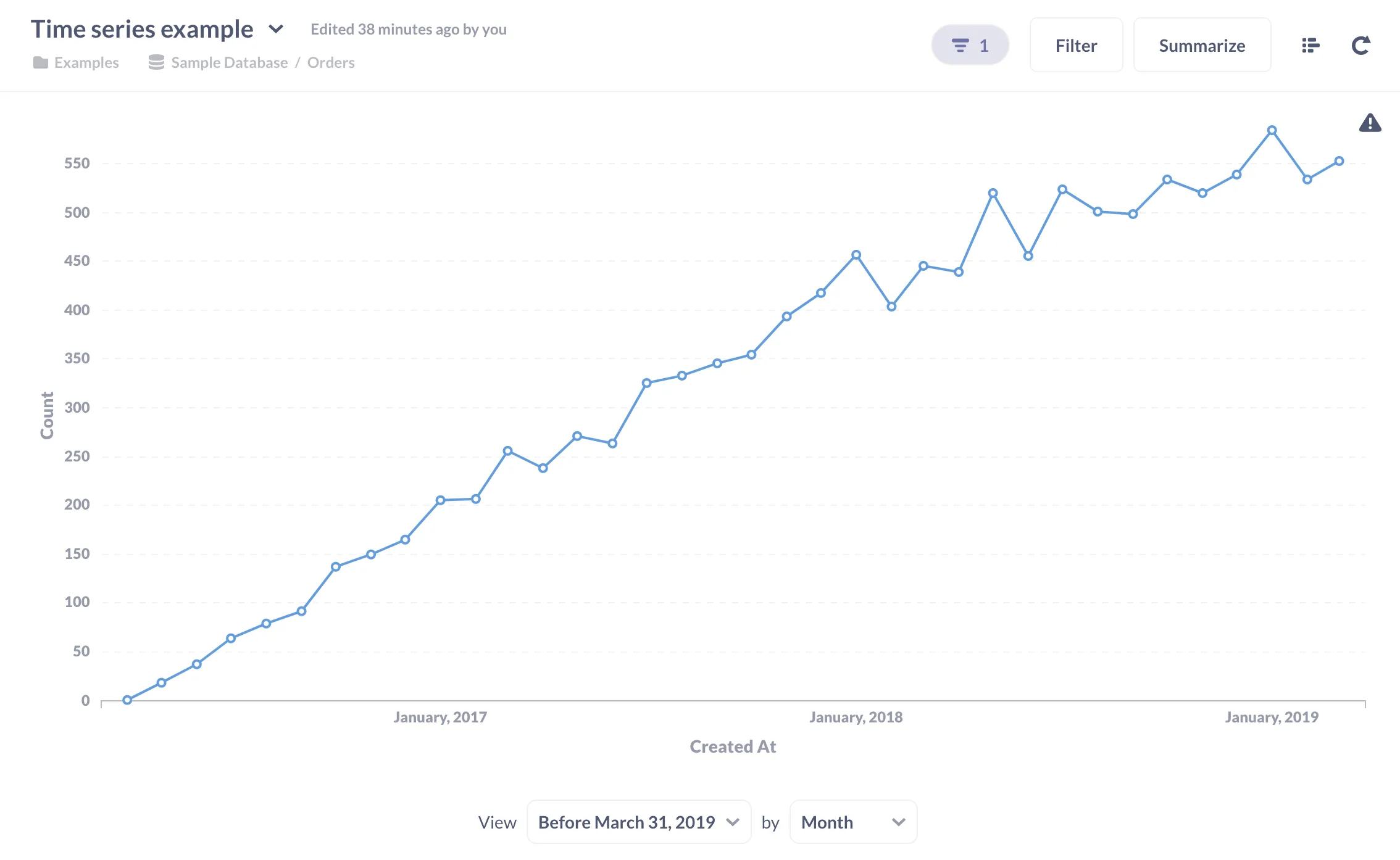# Time series

Also known as

## What is a time series?

A time series is a sequence of data points over time. Times series are used for both analysis (how has the data performed over time) and forecasting (given the line’s trend, what can we expect next week, month, etc.).

Typically you represent a time series as a line chart (called a run chart), but sometimes as a bar chart or area chart. In each case, the horizontal x-axis plots time, and each point plotted on the line is a value at a fixed interval (once an hour, or once a day, etc.). People will use the term “time series” to refer to the actual data by date/time as well as the chart that visualizes that time series.

The values plotted on a time series can be measures (like a temperature) or metrics, such as the total number of orders placed in a week. If you plot the total numbers of orders for each week over fifty-two weeks (or some other period of time), the resulting chart is a visualization of a time series.

## Example time series

Here is a time series of orders per month depicted as a line chart:Fig. 1. A time series showing the count of orders grouped by month.